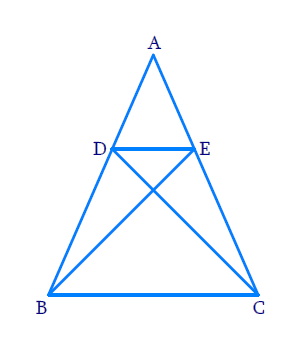# Ex.6.3 Q6 Triangles Solution - NCERT Maths Class 10

Go back to  'Ex.6.3'

## Question

In Figure, if $$\Delta \mathrm{ABE} \cong \Delta \mathrm{ACD}$$, show that $$\Delta ADE \sim \Delta ABC$$.

DiagramVideo Solution
Triangles
Ex 6.3 | Question 6

## Text Solution

Reasoning:

As we know if two triangles are congruent to each other; their corresponding parts are equal.

If one angle of a triangle is equal to one angle of the other triangle and the sides including these angles are proportional, then the two triangles are similar.

This criterion is referred to as the SAS (Side–Angle–Side) similarity criterion for two triangles.

Steps:

In \begin{align}\Delta ABE\,\,{\rm {and}}\,\Delta ACD \end{align}

\begin{align} & AE=AD\\ [ \because \Delta ABE & \cong \Delta ACD \quad (\text{Given})]\ldots (1) \\\\ & AB=AC &\\ [ \because \Delta ABE &\cong \Delta ACD \quad (\text{Given} )]\ldots (2) \\ \end{align}

Now Consider $$\Delta ADE,\,\,\Delta ABC$$

\begin{align} \frac{AD}{AB}&=\frac{AE}{AC}\;\dots\text{from}\,\,(1)\,\And (2)\\\\&\rm{and} \\\\ \angle DAF&=\angle BAC \\(\text{Com}&\text{mon } \text{angle} ) \\\\ \Rightarrow \Delta ADE&\sim{\ }\Delta ABC\\ ( \text{SAS }&\text{criterion} ) \end{align}

Learn from the best math teachers and top your exams

• Live one on one classroom and doubt clearing
• Practice worksheets in and after class for conceptual clarity
• Personalized curriculum to keep up with school